# Does the Cousin Marriage Rate in a Country Have Any Connection with the Type of Alcohol People Like to Drink?

cousins_and_alcohol

# Cousins and Alcohol¶

There is a certain stereotype that says that both alcohol consumption and cousin marriage are bad things, and that they go hand in hand. Regardless of the first part of the stereotype, we can use data to address the second part. Is this just anecdotal or is there a trend in global data that backs this up? To investigate this, we run a simple linear regression between two data sets: the amount and type of alcohol consumed annually per capita by country; and the rate of cousin marriage by country.

The usual caveats apply: this is just using country-wide data and the sources may not have collected it with the same precision in each country. Nevertheless, it is a first step.

First, we'll import the data analysis package pandas for Python. We could just write up the functions themselves (in fact this is done at the end) as they are not going to be very complex, but why re-invent the wheel?

In :
import pandas as pd


Next, we import the two data sets. The first, on cousin marriage, comes from Prof Alan Bittles of Edith Cowan University via fivethirtyeight.com, and can be imported like so:

In :
cousin_df=pd.read_csv('https://raw.githubusercontent.com/fivethirtyeight/data/master/cousin-marriage/cousin-marriage-data.csv')


Note that this is not a list, but a data frame:

In :
type(cousin_df)

Out:
pandas.core.frame.DataFrame

We can peek at the top lines of the data frame with the head() command:

In :
cousin_df.head()

Out:
Country Percent
0 Burkina Faso 65.8
1 Kuwait 51.7
2 Nigeria 51.2
3 Pakistan 51.0
4 Sudan 50.4

Wow, Burkina Faso is way out ahead. Next, we import the other data set on cousin marriage. This comes courtesy of the World Health Organization via fivethirtyeight.com.

In :
alco_df=pd.read_csv('https://raw.githubusercontent.com/fivethirtyeight/data/master/alcohol-consumption/drinks.csv')

In :
alco_df.head()

Out:
country beer_servings spirit_servings wine_servings total_litres_of_pure_alcohol
0 Afghanistan 0 0 0 0.0
1 Albania 89 132 54 4.9
2 Algeria 25 0 14 0.7
3 Andorra 245 138 312 12.4
4 Angola 217 57 45 5.9

Now, a quick glance at both sets shows that there are some naming inconsistencies, e.g. one having the USA under 'United States' and the other under 'USA'. This would be a problem with larger data sets, but we can just do some ad hoc editing here. We will replace 'The Netherlands' by 'Netherlands', 'USA' by 'United States' and 'Great Britian' by 'United Kingdom'. To do this, use the 'replace' function on the dataframe, with a dictionary telling the 'to_replace' command what to do. The second command, inplace='True', tells it to replace the old data frame with the new, correct one.

In :
alco_df['country'].replace(to_replace={'USA':'United States'},inplace=True)


For the other dataframe we must replace two entries:

In :
cousin_df['Country'].replace(to_replace={'The Netherlands':'Netherlands','Great Britain':'United Kingdom'},inplace=True)


Next thing to do is merge both dataframes along the country column. First thing we do is again make a simple edit so that the 'country' in alco_df matches 'Country' (note the capital C!) in cousin_df.

In :
alco_df.rename(columns={'country':'Country'},inplace=True)


Now we are ready to merge the data frames. We use the .join() command.

In :
df=pd.merge(alco_df,cousin_df,on='Country')


Note that this has also discarded any entries with incomplete data, i.e. countries appearing in one data frame but not the other. Next, we import the package statsmodels.api to do the computation of what we are interested in, namely the regression coefficient $\beta_1$ and $R^2.$

In :
import statsmodels.api as sm


We'll also import a graphing package matplotlib.pyplot:

In :
import matplotlib.pyplot as plt

In :
X=df['total_litres_of_pure_alcohol']
Y=df['Percent']


Note that we had to use the function add_constant on X so that the regression line would not be forced to pass through the origin. Next, we specify the kind of model we want (Ordinary Least Squares) and specify the predcitor variable (alcohol consumed) and dependent variable (Percent of Cousin Marriage).

In :
model = sm.OLS(Y,X1).fit()


Finally, we output the stats we are interested in:

In :
model.summary()

Out:
Dep. Variable: R-squared: Percent 0.395 OLS 0.386 Least Squares 44.45 Thu, 25 Apr 2019 5.62e-09 15:52:01 -283.11 70 570.2 68 574.7 1 nonrobust
coef std err t P>|t| [0.025 0.975] 29.6483 2.610 11.360 0.000 24.440 34.856 -2.8982 0.435 -6.667 0.000 -3.766 -2.031
 Omnibus: Durbin-Watson: 23.569 2.113 0 36.986 1.286 9.3e-09 5.463 9.51

Warnings:
 Standard Errors assume that the covariance matrix of the errors is correctly specified.

With $\beta_0$ and $\beta_1$ we can plot the regression (note that we do not include the constant $\beta_0$ in the data, so we use X rather than X1):

In :
plt.plot(X,Y,'.')
f = lambda x : 29.6483 - 2.8982*x
plt.plot(X,f(X), c="red", )
plt.legend()
plt.xlabel('Litres of Alcohol Consumed', fontsize=12)
plt.ylabel('Cousin Marriage Percentage', fontsize=12)
plt.show()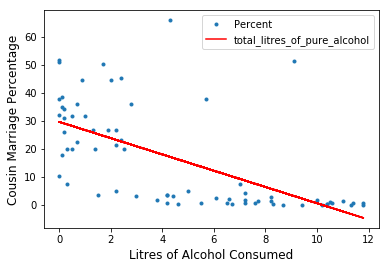Somewhat surprisingly, there is a negative correlation between the amount of alcohol consumed and the prevalence of cousin marriage. In fact, the r-squared statistic implies that almost 40% of the cousin marriage trend can be explained (i.e. correlates with) by lack of alcohol. There could be many intrepretations for this, as well as the possibility that the data itself is not that robust. As we shall see below, it is beer that is best at explaining this effect, although all alcoholic drinks mentioned in the data set explain some of the trend.

We can also ask whether there is significant prediction in the opposite direction: does cousin marriage predict alcohol consumption?

In :
P=df['Percent']
percent_alcohol=sm.OLS(X,P1).fit()
percent_alcohol.summary()

Out:
Dep. Variable: R-squared: total_litres_of_pure_alcohol 0.395 OLS 0.386 Least Squares 44.45 Thu, 25 Apr 2019 5.62e-09 15:52:01 -176.14 70 356.3 68 360.8 1 nonrobust
coef std err t P>|t| [0.025 0.975] 6.8279 0.493 13.844 0.000 5.844 7.812 -0.1364 0.020 -6.667 0.000 -0.177 -0.096
 Omnibus: Durbin-Watson: 3.974 2.055 0.137 3.576 0.554 0.167 3.015 32.8

Warnings:
 Standard Errors assume that the covariance matrix of the errors is correctly specified.
In :
plt.plot(P,X,'.')
f = lambda x : 6.8279 -0.1364*x
plt.plot(P,f(P), c="red")
plt.legend()
plt.xlabel('Cousin Marriage Percentage', fontsize=12)
plt.ylabel('Litres of Alcohol Consumed', fontsize=12)
plt.show()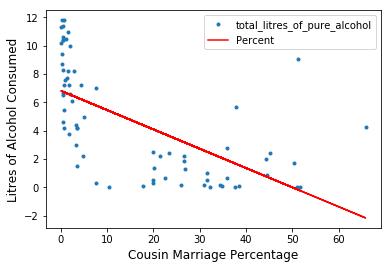Again there is a negative correlation (of course), with the same $R^2$ coefficient.

We now look at the different types of alcohol and see if one is a better predictor of cousin marriage than the others. We start with a linear regression on beer servings as a predictor of cousin marriage:

In :
B=df['beer_servings']
beer_model=sm.OLS(Y,B1).fit()
beer_model.summary()

Out:
Dep. Variable: R-squared: Percent 0.524 OLS 0.517 Least Squares 74.81 Thu, 25 Apr 2019 1.45e-12 15:52:01 -274.75 70 553.5 68 558.0 1 nonrobust
coef std err t P>|t| [0.025 0.975] 29.4190 2.123 13.856 0.000 25.182 33.656 -0.1215 0.014 -8.649 0.000 -0.150 -0.093
 Omnibus: Durbin-Watson: 4.942 1.967 0.085 4.122 0.562 0.127 3.389 216

Warnings:
 Standard Errors assume that the covariance matrix of the errors is correctly specified.
In :
plt.plot(B,Y,'.')
f = lambda x : 29.4190 -0.1215*x
plt.plot(B,f(B), c="red", )
plt.legend()
plt.xlabel('Beer Servings Consumed', fontsize=12)
plt.ylabel('Cousin Marriage Percentage', fontsize=12)
plt.show()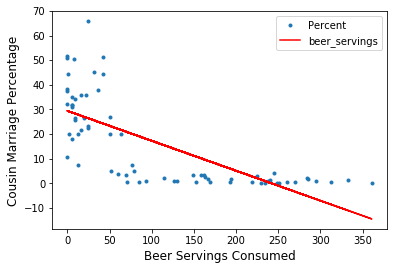This has a higher $R^2$, meaning that annual beer servings per capita explain about 52% of the drop off in cousin marriage as annual beer servings per capita increase. So beer annual servings per capita better explain the lack of cousin marriage than annual litres of alcohol consumed per capita, according to this data set.

While the $\beta_1$ slope coefficient of the regression line is smaller than the slope coefficient for the total amount of alcohol consumed, this is mainly due to the change in units, since one serving of beer only contains about 0.018 litres of pure alcohol (if the beer has 5% ABV). Larger numbers for the predictor would therefore mean a smaller slope if the dependent variable's units are held fixed. Comapring the graphs, the slopes look very similar because they have been resized to fit all data points, compensating for the change of units.

Conversely, we get a similar negative correlation for cousin marriage as a predictor of beer consumption:

In :
percent_beer=sm.OLS(B,P1).fit()
percent_beer.summary()

Out:
Dep. Variable: R-squared: beer_servings 0.524 OLS 0.517 Least Squares 74.81 Thu, 25 Apr 2019 1.45e-12 15:52:02 -399.66 70 803.3 68 807.8 1 nonrobust
coef std err t P>|t| [0.025 0.975] 178.2149 12.017 14.831 0.000 154.236 202.194 -4.3109 0.498 -8.649 0.000 -5.305 -3.316
 Omnibus: Durbin-Watson: 2.637 2.091 0.268 2.437 0.373 0.296 2.473 32.8

Warnings:
 Standard Errors assume that the covariance matrix of the errors is correctly specified.
In :
plt.plot(P,B,'.')
f = lambda x : 178.2149 -4.3109*x
plt.plot(P,f(P), c="red")
plt.legend()
plt.xlabel('Cousin Marriage Percentage', fontsize=12)
plt.ylabel('Beer Servings Consumed', fontsize=12)
plt.show()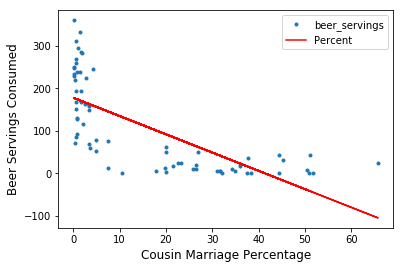Next up, to what extent do annual spirit servings per capita predict the prevalence of cousin marraige?

In :
S=df['spirit_servings']
spirit_model=sm.OLS(Y,S1).fit()
spirit_model.summary()

Out:
Dep. Variable: R-squared: Percent 0.309 OLS 0.299 Least Squares 30.46 Thu, 25 Apr 2019 5.76e-07 15:52:02 -287.76 70 579.5 68 584.0 1 nonrobust
coef std err t P>|t| [0.025 0.975] 27.6759 2.729 10.140 0.000 22.230 33.122 -0.1660 0.030 -5.519 0.000 -0.226 -0.106
 Omnibus: Durbin-Watson: 4.567 2.073 0.102 4.552 0.603 0.103 2.672 138

Warnings:
 Standard Errors assume that the covariance matrix of the errors is correctly specified.
In :
plt.plot(S,Y,'.')
f = lambda x : 27.6759 -0.1660*x
plt.plot(S,f(S), c="red", )
plt.legend()
plt.xlabel('Spirit Servings Consumed', fontsize=12)
plt.ylabel('Cousin Marriage Percentage', fontsize=12)
plt.show()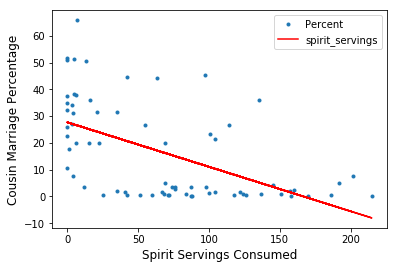Here, the $R^2$ is smaller than that of the overall alcohol consumed, meaning that it is not as good a predictor as overall alcohol, and certainly much less than beer. Nevertheless, it does explain about 31% of the decline in the prevalence of cousin marriage as annual servings of spirits per capita increases.

In :
percent_spirits=sm.OLS(S,P1).fit()
percent_spirits.summary()

Out:
Dep. Variable: R-squared: spirit_servings 0.309 OLS 0.299 Least Squares 30.46 Thu, 25 Apr 2019 5.76e-07 15:52:03 -372.41 70 748.8 68 753.3 1 nonrobust
coef std err t P>|t| [0.025 0.975] 98.9043 8.142 12.147 0.000 82.656 115.152 -1.8639 0.338 -5.519 0.000 -2.538 -1.190
 Omnibus: Durbin-Watson: 4.204 1.815 0.122 4.139 0.558 0.126 2.584 32.8

Warnings:
 Standard Errors assume that the covariance matrix of the errors is correctly specified.
In :
plt.plot(P,S,'.')
f = lambda x : 98.9043 -1.8639*x
plt.plot(P,f(P), c="red")
plt.legend()
plt.xlabel('Cousin Marriage Percentage', fontsize=12)
plt.ylabel('Spirit Servings Consumed', fontsize=12)
plt.show()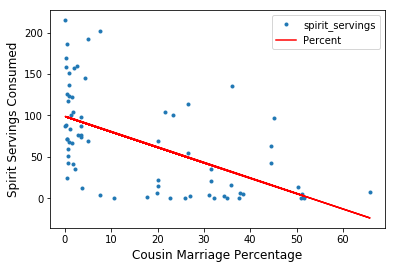Finally, annual wine servings per capita as a predictor of cousin marriage:

In :
W=df['wine_servings']
wine_model=sm.OLS(Y,W1).fit()
wine_model.summary()

Out:
Dep. Variable: R-squared: Percent 0.278 OLS 0.268 Least Squares 26.22 Thu, 25 Apr 2019 2.70e-06 15:52:03 -289.30 70 582.6 68 587.1 1 nonrobust
coef std err t P>|t| [0.025 0.975] 22.4169 2.185 10.261 0.000 18.057 26.776 -0.0981 0.019 -5.120 0.000 -0.136 -0.060
 Omnibus: Durbin-Watson: 3.51 2.01 0.173 3.427 0.526 0.18 2.739 136

Warnings:
 Standard Errors assume that the covariance matrix of the errors is correctly specified.
In :
plt.plot(W,Y,'.')
f = lambda x : 22.4169 -0.0981*x
plt.plot(W,f(W), c="red", )
plt.legend()
plt.xlabel('Wine Servings Consumed', fontsize=12)
plt.ylabel('Cousin Marriage Percentage', fontsize=12)
plt.show()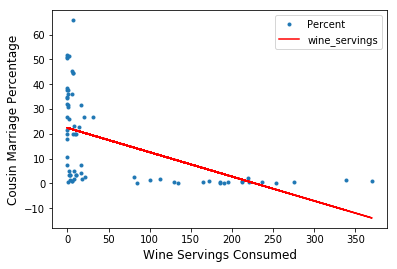This has the worst $R^2$ of all, but at nearly 28% is still not terrible. Wine is the worst at predicting this trend.

In :
percent_wine=sm.OLS(W,P1).fit()
percent_wine.summary()

Out:
Dep. Variable: R-squared: wine_servings 0.278 OLS 0.268 Least Squares 26.22 Thu, 25 Apr 2019 2.70e-06 15:52:03 -407.06 70 818.1 68 822.6 1 nonrobust
coef std err t P>|t| [0.025 0.975] 108.5659 13.357 8.128 0.000 81.912 135.220 -2.8368 0.554 -5.120 0.000 -3.942 -1.731
 Omnibus: Durbin-Watson: 12.25 2.401 0.002 12.717 0.956 0.00173 3.839 32.8

Warnings:
 Standard Errors assume that the covariance matrix of the errors is correctly specified.
In :
plt.plot(P,W,'.')
f = lambda x : 108.5659 -2.8368*x
plt.plot(P,f(P), c="red")
plt.legend()
plt.xlabel('Cousin Marriage Percentage', fontsize=12)
plt.ylabel('Wine Servings Consumed', fontsize=12)
plt.show()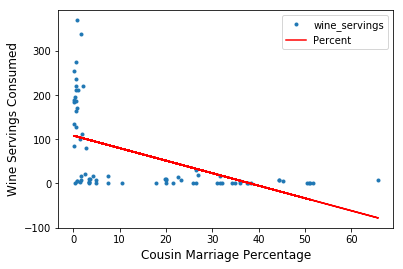##### For those interested, the regression in the table above can be calculated 'by hand' below.¶

First, this function gives the regression coefficients, i.e. the $y$-intercept $\beta_0$ and the line's slope $\beta_1$:

In :
def lin_reg(L):
x_sq=[L[i]**2 for i in range(0,len(L))]
xy=[L[i]*L[i] for i in range(0,len(L))]
x=[L[i] for i in range(0,len(L))]
y=[L[i] for i in range(0,len(L))]
n=len(L)
X=sum(x)/n
Y=sum(y)/n
XY=sum(xy)/n
X2=sum(x_sq)/n
b0=(X*XY-X2*Y)/(X**2-X2)
b1=(X*Y-XY)/(X**2-X2)
return b0,b1


Next we have the Mean Squared Error, sample variance, $R^2$ coefficient of determination and Pearson correlation coefficient:

In :
def MSE(L):
L=[(L[i]-lin_reg(L)-lin_reg(L)*L[i])**2 for i in range(0,len(L))]
return sum(L)/(len(L)-2)

In :
def sample_variance(L):
n=len(L)
A=[L[i] for i in range(0,n)]
av=sum(A)/n
L=[(L[i]-av)**2 for i in range(0,n)]
return (sum(L)/(n-1))**0.5

In :
def r_squared(L):
n=len(L)
b0=lin_reg(L)
b1=lin_reg(L)
Y_hat=[b0+b1*L[i] for i in range(0,n)]
A=[L[i] for i in range(0,n)]
avg=sum(A)/n
Sq=[(a-avg)**2 for a in A]
SSTO=sum(Sq)
return 1-MSE(L)*(n-2)/SSTO

In :
def pearson(L):
if lin_reg(L)>0:
return r_squared(L)**0.5
else:
return -r_squared(L)**0.5


To run all these, we need to convert our dataframe into a list (of lists), which only contains the predictors and dependent variables:

In :
D=df.values.tolist()
L=[[d[-2],d[-1]] for d in D]
BL=[[d,d[-1]] for d in D]
SL=[[d,d[-1]] for d in D]
WL=[[d,d[-1]] for d in D]


First, we get the linear regression coefficients:

In :
print(lin_reg(L))
print(lin_reg(BL))
print(lin_reg(SL))
print(lin_reg(WL))

(29.64827773417355, -2.8981676741922073)
(29.419044340941255, -0.12151331619667606)
(27.675867764348144, -0.16597388313268766)
(22.416865032793417, -0.09809274468031609)


Next, the mean squared error and sample variance (these are not in the table above):

In :
print(MSE(L))
print(MSE(BL))
print(MSE(SL))
print(MSE(WL))

196.37035375828748
154.62146332410288
224.26450303867117
234.362027070373

In :
print(sample_variance(L))

17.888968633148306


Note that this calculates the variance in the cousin marriage data only, and be the same for all the alcohol data sets.

Finally, the $R^2$ and Pearson coefficient:

In :
print(r_squared(L),pearson(L))
print(r_squared(BL),pearson(BL))
print(r_squared(SL),pearson(SL))
print(r_squared(WL),pearson(WL))

0.39526495193480204 -0.6287010036056901
0.5238333268457211 -0.7237633085793457
0.3093631374146012 -0.5562042227586925
0.2782671671533927 -0.5275103479111976